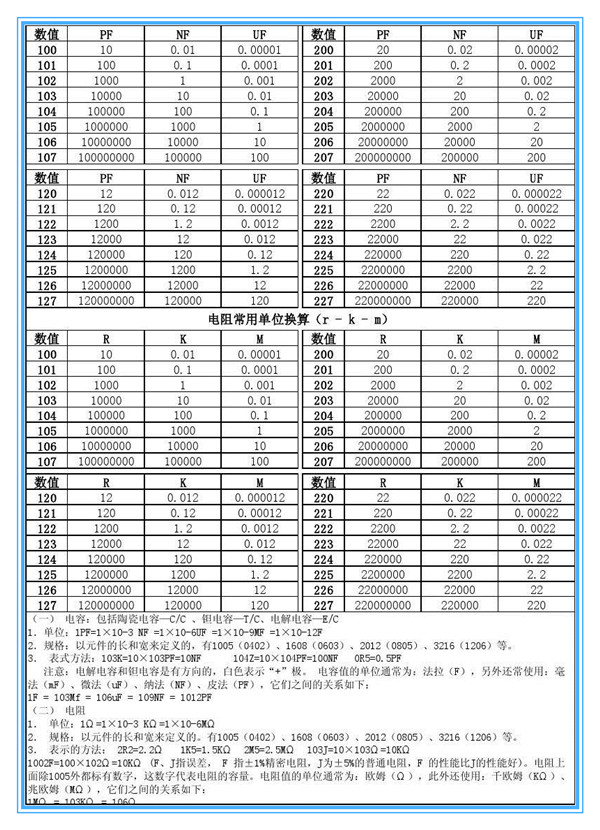# 电容的单位换算

1F=10^6uF=10^9nF=10^12pF

1F=10^6uF=10^12pF
1F＝1000000µF

105= 1 µF=1000nF=1000000pF
104= 0.1 µF
103= 0.01 µF=10000PF
102= 0.001 µF =1000PF
224=0.22uFC＝P/2πfU2(tgφ1－tgφ)

U－系统电压，单位是伏；
F－系统频率，单位是赫；
φ1－并联电容之前，负载的阻抗角；
φ－并联电容之后，系统的阻抗角； C－补偿电容，单位是法。 进口电容的标识,基本单位,单位换算关系 <1>单位：基本单位为P，辅助单位有G，M，N。换算关系为：<1G=1000µF><1M=1µF=1000PF> <2>标注法：通常不是小数点，而是用单位整数，将小数部分隔开。